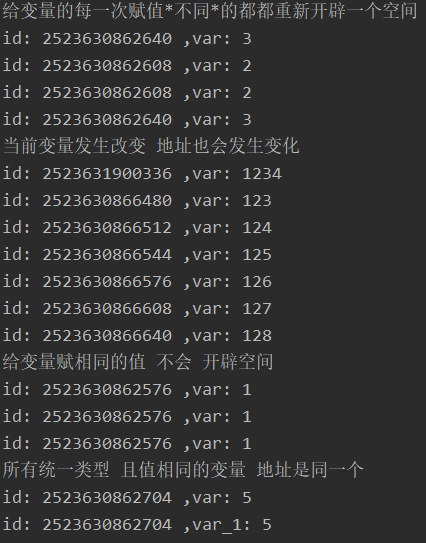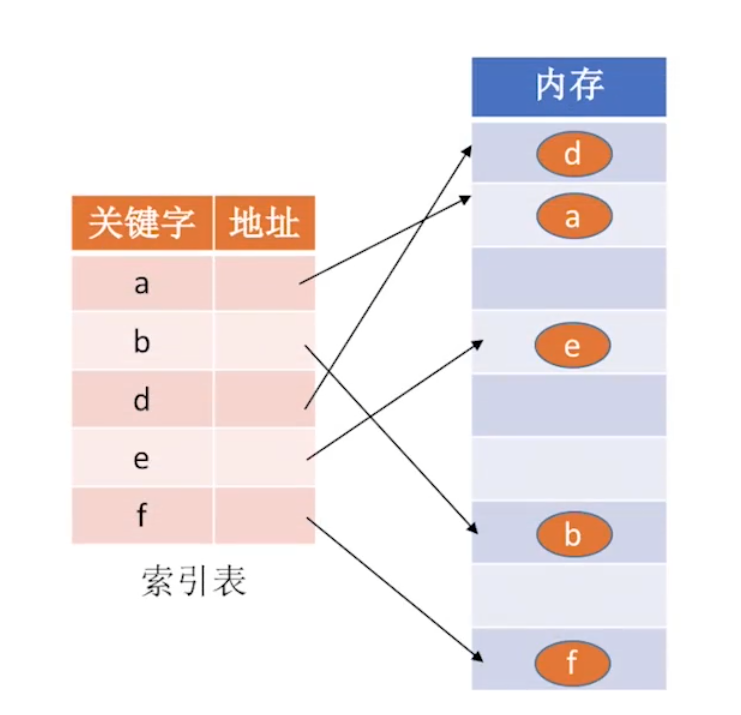//这种时候一般会定义两个指向同一个开辟的内存空间的指针变量，一个用于操作，一个用于释放，避免造成内存泄漏
char *p = (char *)malloc(100);
strcpy(p, "abcdefg");
p += 1; //对指针指向进行改变操作
*p = 'a';
free(p);
p = NULL;


C语言没有垃圾回收机制，内存的开辟和回收都是自己来控制的，如果指针使用不当很容易造成内存泄漏。

## 值类型 引用类型变量

• 值类型（无法修改开辟空间对应的地址数据）：字符串、元组、数子 布尔

• 引用类型：列表、字典, 集合 字符串

### 值类型

1. 开辟内存(声明),将4存储到该内存中（赋值）
2. a仅仅代表该变量地址的别名，谁会愿意记住一串地址呢
3. 再次赋值都是在该段内存中继续操作

1. 在这里变量无需声明，直接可以拿来使用。或者可以说赋值和声明操作时一体的赋值的同时就在声明。如果一个变量没有赋值那么，我们也就无法对他操作。
2. 严格在某个方面来说没有变量类型这个概念
3. 每一个变量就和C语言的指针类似，站在c语言的角度看可以说变量可以是可以在使用时自动强制转换的指针，该变量就是解地址

1. 检查 4 这个 int 对象是否在内存中存在，如果存在执行2，不存在执行3
2. 将var指向4
3. 开辟一段内存，存储4，将4指向var

import random
# 如果一个变量没有赋值那么，我们也就无法对他操作。
# print("id:" % id(var))

print("给变量的每一次赋值*不同*的都都重新开辟一个空间")
for i in range(4):
var = random.randint(0, 3)
print("id: %d ,var: %s" % (id(var), var))

print("当前变量发生改变 地址也会发生变化")
var = 1234
print("id: %d ,var: %s" % (id(var), var))
var = 123
print("id: %d ,var: %s" % (id(var), var))
for i in range(5):
var += 1
print("id: %d ,var: %s" % (id(var), var))

print("给变量赋相同的值 不会 开辟空间")
for i in range(3):
var = 1
print("id: %d ,var: %s" % (id(var), var))

print("所有统一类型 且值相同的变量 地址是同一个")
var = 5
var_1 = 5
print("id: %d ,var: %s" % (id(var), var))
print("id: %d ,var_1: %s" % (id(var_1), var_1))#引用类型list1 = [1, 2, 3, 4]
list2 = list1
list2 = 3
print(list1)


list2 = list1，就好比把list1的地址给了 list2

list1 = [1, 2, 3, 4]
list2 = list1
list2 = [1, 2]
print(list1)


list2 = [1, 2]就是把list2指向了一个新内存地址，该内存段存着 [1,2]

## 结语

Q.E.D.

••